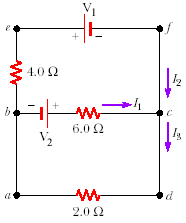# Kirchhoff's Rules Circuit Problem

## Homework Statement

Where V1 = 14V and V2 = 10V, solve for I1, I2, and I3.## Homework Equations

DeltaV = IR
Sigma(I) at junction = 0
Sigma(DeltaV) of loop = 0

## The Attempt at a Solution

I began by assuming clockwise direction of the loops, to determine the signs of the resistance to follow. I planned to solve by using the following three equations:
I3 = I1 + I2
(Upper loop) -14V - 10V + 6I1 - 4I2 = 0
(Lower loop) 10V - 2I3 - 6I1
I began by solving for I1 using the lower loop equation, resulting in I1 = 1.67 - 0.33I3
I plugged in the solution for I1 into the upper loop equation to solve for I2 in terms of I3, resulting in I2 = -8.5 - 0.5I3
I then plugged in the solutions for I2 and I1 into the first equation to solve for I3, which resulted in I3 = -3.7
The correct value for I3 should be -1A.

I know the equations I am starting with are correct, according to the answer. I don't understand where I'm going wrong with the algebra. It all looks like it should be work to me.

Last edited:
•fireflies

SteamKing
Staff Emeritus
Homework Helper
The direction shown for the current I1 doesn't seem to be consistent with the assumption that the loop currents travel in a clockwise direction.

•fireflies
The clockwise direction traversed is just used to find the signs of each resistance. If the current with a resistor goes with the clockwise direction previously chosen for the loop, the resistor has a negative voltage. If the current with a resistor goes opposite the clockwise direction chosen for the loop, the resistor has a positive voltage. This is why the upper loop equation is adding the 6I1 and subtracting the 4I2. They move in opposite directions within the upper loop, and a clockwise or counter clockwise direction is chosen to be consistent with signs within the loop, but from what I was taught, either direction can be chosen as long as you stick with it.

The equations used at the top of my attempt are absolutely correct according to the book. When solving for the variables is where I'm going wrong somehow. I don't understand why the algebra I'm doing is incorrect.

SteamKing
Staff Emeritus
Homework Helper
The clockwise direction traversed is just used to find the signs of each resistance. If the current with a resistor goes with the clockwise direction previously chosen for the loop, the resistor has a negative voltage. If the current with a resistor goes opposite the clockwise direction chosen for the loop, the resistor has a positive voltage. This is why the upper loop equation is adding the 6I1 and subtracting the 4I2. They move in opposite directions within the upper loop, and a clockwise or counter clockwise direction is chosen to be consistent with signs within the loop, but from what I was taught, either direction can be chosen as long as you stick with it.

The equations used at the top of my attempt are absolutely correct according to the book. When solving for the variables is where I'm going wrong somehow. I don't understand why the algebra I'm doing is incorrect.
Double check where you substitute I1 = 1.67 - 0.33 I3 into your Upper Loop equation. I think you've made an arithmetic mistake there.

6I1 = 10 - 2I3, according to your third equation.

•scharry03
YES you are absolutely right, I accidentally made the 6 x 1.67 a negative, not a positive!! I got the right answer now, thanks so much!